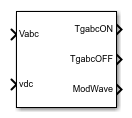# PWM Timing and Waveform Generator (Three-phase, Two-level)

Generate three-phase, two-level gating time information

• Library:
• Simscape / Electrical / Control / Pulse Width Modulation

•## Description

The PWM Timing and Waveform Generator (Three-phase, Two-level) block controls switching behavior for a three-phase, two-level power converter. The block calculates on-gating and off-gating times and modulation waves based on these block inputs:

• Three sinusoidal reference voltages

This block can be used with average converters to speed up the simulation.

### Continuous and Discontinuous PWM

The block provides modes for both continuous and discontinuous pulse width modulation (PWM). The figure shows the general difference between continuous sinusoidal pulse width modulation (SPWM) and continuous space vector modulation (SVM) waveforms.For discontinuous pulse width modulation (DPWM), the block clamps the modulation wave to the positive or negative DC rail for a total of 120 degrees during each fundamental period. During the clamping intervals, modulation discontinues.

A waveform with 30-degree DPWM has four 30-degree intervals per fundamental period.Selecting a positive or negative 30-degree phase shift affects the clamping intervals for 60-degree DPWM.The figure shows the waveforms for positive and negative DC clamping for 120-degree DPWM.### Sampling Mode

This block allows you to choose natural, symmetric, or asymmetric sampling of the modulation wave.

The PWM Timing and Waveform Generator (Three-phase, Two-level) block does not perform carrier-based PWM. Instead, the block uses input signals to calculate the gating times.

Carrier-based PWM is, however, useful for showing how the sampling mode that you select relates to the switch-on and switch-off behavior of the pulses that the block generates. A generator that uses a two-level, carrier-based PWM method:

1. Samples a reference wave

2. Compares the sample to a triangle carrier wave

3. Generates a switch-on pulse if a sample is higher than the carrier signal or a switch-off pulse if a sample is lower than the carrier wave

To determine switch-on and switch-off pulse behavior, a two-level carrier-based PWM generator uses these methods to sample the triangle wave:

• Natural — The sampling and comparison occur at the intersection points of the modulation wave and the carrier wave.• Asymmetric — Sampling occurs at the upper and lower boundaries of the carrier wave. The comparison occurs at the intersection that follows the sampling.• Symmetric — Sampling occurs at only the upper boundary of the carrier wave. The comparison occurs at the intersection that follows the sampling.## Ports

### Input

expand all

Sinusoidal voltages that you want the attached converter to output, specified as a vector of three elements, with one element for each phase.

Positive real number for the DC-link voltage of the converter, specified as a scalar.

### Output

expand all

Switch-on gating time, returned as a vector of three elements, with one element for each phase.

Switch-off gating time, returned as a vector of three elements, with one element for each phase.

Modulation waves, returned as a vector of three elements, with one element for each phase.

## Parameters

expand all

Pulse width modulation method.`Discontinuous PWM (DPWM)` clamps the waveform to the DC rail for a total of 120 degrees in each fundamental period. `Continuous PWM (CPWM)` does not.

Continuous pulse width modulation method.

#### Dependencies

To enable this parameter, set PWM mode to `Continuous PWM (CPWM)`.

Discontinuous pulse width modulation method. Specify the method for distributing the 120 degrees per period during which the block clamps the modulation wave to the DC rail. Options include:

• ```60 DPWM: 60 degree discontinuous PWM```

• ```60 DPWM (+30 degree shift): +30 degree shift from 60 DPWM```

• ```60 DPWM (-30 degree shift): -30 degree shift from 60 DPWM```

• ```30 DPWM: 30 degree discontinuous PWM```

• ```120 DPWM: positive dc component```

• ```120 DPWM: negative dc component```

When the wave is clamped, modulation discontinues.

#### Dependencies

To enable this parameter, set PWM mode to `Discontinuous PWM (DPWM)`.

Wave-sampling method. The sampling mode determines whether the block samples the modulation waveform when the waves intersect or when the carrier wave is at one or both of its boundary conditions.

Rate at which the switches in the power converter switch.

 Chung, D. W., J. S. Kim, and S. K. Sul. “Unified Voltage Modulation Technique for Real Time Three-Phase Power Conversion.” IEEE Transactions on Industry Applications, Vol. 34, No. 2, 1998, pp. 374–380.

 Seo, J. H., C. H. Choi, and D. S. Hyun. “A new simplified space-vector PWM method for three-level inverters.” IEEE Transactions on Power Electronics, Vol. 16, No. 4, 2001, pp. 545-550.